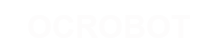#### 站点工具

reference:language:if

## if（条件判断语句）和 ==、!=、<、>（比较运算符）

if 语句与比较运算符一起用于检测某个条件是否达成，如某输入值是否在特定值之上等。if 语句的语法是：

```if (someVariable > 50)
{
// 执行某些语句
}```

```if (x > 120) digitalWrite(LEDpin, HIGH);

if (x > 120)
digitalWrite(LEDpin, HIGH);

if (x > 120){ digitalWrite(LEDpin, HIGH); }

if (x > 120){
digitalWrite(LEDpin1, HIGH);
digitalWrite(LEDpin2, HIGH);
}                                 // 以上所有书写方式都正确```

#### 比较运算操作符：

``` x == y（x 等于 y）
x != y（x 不等于 y）
x <  y（x 小于 y）
x >  y（x 大于 y）
x <= y（x 小于等于 y）
x >= y（x 大于等于 y）```

#### 警告：

if 的另外一种分支条件控制结构是 if...else 形式。

reference/language/if.txt · 最后更改: 2017/04/07 10:03 (外部编辑)

### 页面工具